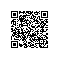# 男生追女生的超强数学建模分析

1、t时刻A君的学业成绩为Y(t)；
2、t时刻B女对A君的疏远度为X(t)；
3、当A君没开始追求B女时B女对A君的疏远度增长（平时发现的A君的不良行为）符合Malthus模型，即dX/dt=aX(t)其中a为正常数。
4、当Y(t)存在时，单位时间内减少X(t)的值与X(t)的值成正比，比例常数为b，从而 dX(t)/dt=aX(t)-bX(t)Y(t)。
5、A君发起对B女追求后，立即转化为B女对A君的好感，并设定转化系数为 α，而随着的A君发起对B女的追求，A君学业的自然下降率与学业成绩成正比，比例系数为e。于是有dY(t)/dt=αbX(t)Y(t)-eY(t)。

{dX(t)/dt=aX-bXY；dY(t)/dt=cXY-eY} 其中c=αb. (1)

F(X,Y)=cX-dln|X|-aln|Y|=k (2)

∫(dy/Ydt)dt=c∮Xdt-dT (3)

{dX/dT=aX-bXY-hX=(a-h)X-bXY；dY/dt=cXY-eY-hY=cXY-(e+h)Y} (4)

x’=(∫Xdt)/T=(e+h)/c，y’=(∫Ydt)/t=(a-h)/b (5)使用钉钉扫一扫加入圈子
+ 订阅